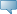## Catalog Entries

Fall 2020
Oct 01, 2022Select the link beside Class Schedule to find available classes for the course.

 MATH 3132 - Engineering Mathematical Analysis 3 (Lab required) Vector integral calculus; series of Ordinary differential equations; Fourier series and Partial differential equations. For Engineering and Geophysics students only. May not be held with the former MATH 3740, the former MATH 3800, or the former MATH 3100. Prerequisites: MATH 2130 and MATH 2132. - 3.0 Credit hours Science, Science lab Mathematics Department Course Attributes: Mathematics Requirement, Science Requirement for BA, Science